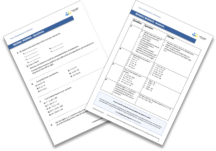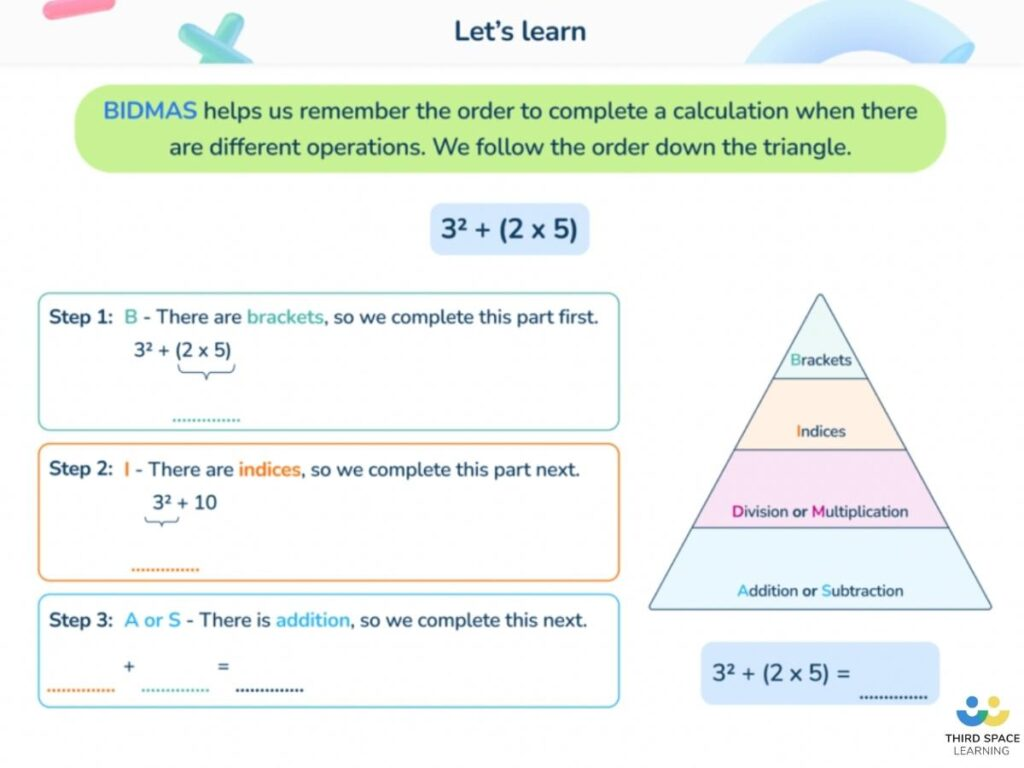Teaching support from the UK’s largest provider of in-school maths tuitionone to one lessonsschools supported

Consolidate learning and plug gaps

In-school online one to one maths tuition developed by maths teachers and pedagogy experts

See it in action

Hundreds of FREE online maths resources!

Daily activities, ready-to-go lesson slides, SATs revision packs, video CPD and more!Explore all resources
Four Operations

Consolidate learning and plug gaps

In-school online one to one maths tuition developed by maths teachers and pedagogy experts

See it in action

# What is BODMAS And BIDMAS: Explained For Primary School Parents With Practice Questions

BODMAS and BIDMAS crop up throughout primary school maths so here we cover what BODMAS/BIDMAS mean, and provide you with some BODMAS questions and exercises you can use to help your KS2 child practise accurately carrying out BODMAS calculations.

### What is BODMAS?

BODMAS is an acronym to help children remember the order of mathematical operations – the correct order in which to solve maths problems.

Bodmas stands for B-Brackets, O-Orders (powers/indices or roots), D-Division, M-Multiplication, A-Addition, S-Subtraction.

### What is the BODMAS rule

The Bodmas rule states that division and multiplication must be done before addition and subtraction in any mathematical example. And if there are brackets (or parentheses) and orders (of powers or roots), these must be done first of all. For example in the question 47 + 54 ÷ 9 the answer is 53 not 11.2r.

The Bodmas rule follows the order of the BODMAS acronym ie  B – Brackets, O – Order of powers or roots, D – Division, M – Multiplication A – Addition, and S – Subtraction. Mathematical expressions with multiple operators need to be solved from left to right in the order of BODMAS. Division and Multiplication are considered as interchangeable and depend on which comes first in the expression, as are Addition and Subtraction.

Some children use the Bodmas rule as a mnemonic (like Richard Of York Gave Battle In Vain is used to remember the colours Red, Orange, Yellow, Green, Blue, Indigo, Violet).

### What is BIDMAS?

The BIDMAS rule is an alternative acronym to BODMAS to help remember the order of operations. The only difference is that there’s an I instead of O. The meaning is the same. Bidmas is the term more commonly used in primary schools today.

Bidmas stands for Brackets, Indices, Division/Multiplication, Addition/Subtraction.

### Mathematical Operations

“Mathematical operations” are what you do to the numbers given. The four main operations are:

• subtraction (-);
• multiplication (x);
• and division (÷).Year 6 BIDMAS or BODMAS Worksheet

Download this FREE worksheet, that includes 14 questions to practise working with BIDMAS and BODMAS.Download Free Now!

### BODMAS meaning

When presented with a number sentence containing more than one operation (such as 3 + 4 x 2) the operations cannot be completed from left to right, but instead in their order of “importance”, which is what BODMAS stands for.

BODMAS stands for:

Brackets
Orders
Division/Multiplication

“Orders” means square roots and indices (which you may know as square numbers, powers or exponents).

### BIDMAS meaning

Brackets
Indices
Division/Multiplication

Here “Indices” (square numbers, powers or exponents) are used instead of Orders.

BODMAS and BIDMAS can also be referred to as PEMDAS.

### What does order of operations mean?

This is the order in which certain operations must be completed, from brackets (also known as parentheses) first to addition and subtraction last.

It is important that division and multiplication are represented alongside each other as they are of equal importance (so must be completed from left to right, whichever appears first) – this is the same for addition and subtraction.

### BODMAS examples

Below are some examples of BODMAS questions and answers children might see in schools. We’ve given you the right answer and at least one different answer to show you where children might go wrong.

### BODMAS (BIDMAS) Questions and Answers

Question 1: 6 + 2 x 7

The multiplication must be completed first (2 x 7 = 14) and then the addition (6 + 14 = 20).

This may be commonly miscalculated as 56 by working from left to right (6 + 2 = 8, 8 x 7 = 56).

Question 2: 3 x (2 + 4) + 52

The BODMAS rule states we should calculate the Brackets first (2 + 4 = 6), then the Orders (52 = 25), then any Division or Multiplication (3 x 6 (the answer to the brackets) = 18), and finally any Addition or Subtraction (18 + 25 = 43).

Children can get the wrong answer of 35 by working from left to right.

Question 3: 5 – 2 + 6 ÷ 3

The division must be completed first (6 ÷ 3 = 2) which then leaves addition and subtraction; as both are of the same importance, we can then work from left to right. 5 – 2 + 2 (the answer to 6 ÷ 3) = 5.

This may be commonly miscalculated as either 3 by working from left to right, or as 1 by wrongly assuming that addition should be completed before subtraction.

### When will my child learn about BODMAS in primary school?

BODMAS is taught in upper KS2 and often primary school children won’t come across the order of operations until Year 6.

The national curriculum states that Year 6 pupils should be taught to use their knowledge of the order of operations to carry out calculations involving the four operations.

The non-statutory guidance advises that pupils explore the order of operations using brackets; for example, 2 + 1 x 3 = 5 and (2 + 1) x 3 = 9.Teaching BIDMAS and the order of operations is something that the tutors from Third Space Learning are very familiar.

### BODMAS calculator

As a parent trying to support your child with order of operations questions you’ll find that most calculators and computers nowadays are sophisticated enough to complete calculations according to BODMAS. However it’s worth testing any calculator out just to be sure. There are also plenty of BODMAS calculators available online.

### Practice KS2 BODMAS questions

1) 29 – 4 x 6 + 5 =

2) Write what the two missing numbers could be. (4 + ?) x ? = 100

Answer: 6 and 10 (4 + 6) x 10 = 100

3) Write the missing numbers to make these calculations correct.

a) 200 x ? – 200 = 200

b) (100 – ?) x 100 = 100

4) Write the correct sign >, < or = in each of the following

a) (10 + 5) – 9 [     ] (10 + 9) – 5

b) 3 x (4+5) [     ]  (3 x 4) + 5

c) (10 x 4) / 2 [     ]  10 x (4 / 2)

a) (10 + 5) – 9 < (10 + 9) – 5

b) 3 x (4+5) > (3 x 4) + 5

c) (10 x 4) / 2 = 10 x (4 / 2)

### Practice Year 6 BODMAS questions

#### Other tricky primary maths curriculum topics you may need help on

If you feel a child needs some extra support to understand BODMAS, the order of operations, or the other trickier aspects of the primary maths curriculum, Third Space Learning’s online maths lessons provide 1-to-1 support that’s personalised to children’s needs. Tutors develop confidence, and make lessons fun, matching the interests and previous maths experience of the child being taught.

Online 1-to-1 maths lessons trusted by schools and teachers
Every week Third Space Learning’s maths specialist tutors support thousands of pupils across hundreds of schools with weekly online 1-to-1 lessons and maths interventions designed to plug gaps and boost progress.

Since 2013 we’ve helped over 80,000 primary and secondary school pupils become more confident, able mathematicians. Learn more or request a personalised quote to speak to us about your school’s needs and how we can help.

Primary school tuition targeted to the needs of each child and closely following the National Curriculum.##### Ellie Williams
Third Space Learning
Content Team
With a love for all things KS2 maths, Ellie is a part of the content team that helps all of the Third Space Learning blogs and resources reach teachers!

Related Articles

x#### Year 6 BIDMAS or BODMAS Worksheet

Download this FREE worksheet, that includes 14 questions to practise working with BIDMAS and BODMAS.

#### Year 6 BIDMAS or BODMAS WorksheetDownload this FREE worksheet, that includes 14 questions to practise working with BIDMAS and BODMAS.